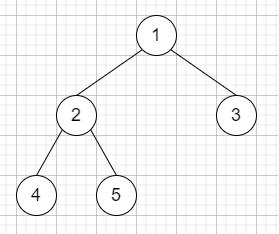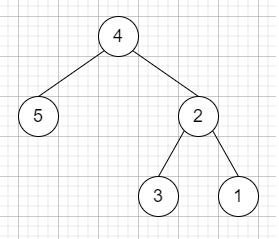# Binary Tree Upside Down in C++

Suppose we have a binary tree where all the right nodes are either leaf nodes with a sibling otherwise empty, we have to flip it upside down and turn it into a tree where the original right nodes turned into left leaf nodes. We have to return the new node.

So, if the input is like [1,2,3,4,5]then the output will return the root of the binary tree [4,5,2,#,#,3,1]To solve this, we will follow these steps −

• Define a function solve(), this will take node, par, sibling,

• if node is not present, then −

• return NULL

• child = left of node

• currSib = right of node

• node := left of sibling

• node := right of par

• if child and currSib is not present, then −

• return node

• return solve(child, node, currSib)

• From the main method do the following −

• return solve(root, NULL, NULL)

## Example

Let us see the following implementation to get better understanding −

Live Demo

#include <bits/stdc++.h>
using namespace std;
class TreeNode{
public:
int val;
TreeNode *left, *right;
TreeNode(int data){
val = data;
left = NULL;
right = NULL;
}
};
void insert(TreeNode **root, int val){
queue q;
q.push(*root);
while(q.size()){
TreeNode *temp = q.front();
q.pop();
if(!temp->left){
if(val != NULL)
temp->left = new TreeNode(val);
else
temp->left = new TreeNode(0);
return;
}
else{
q.push(temp->left);
}
if(!temp->right){
if(val != NULL)
temp->right = new TreeNode(val);
else
temp->right = new TreeNode(0);
return;
}
else{
q.push(temp->right);
}
}
}
TreeNode *make_tree(vector<int< v){
TreeNode *root = new TreeNode(v);
for(int i = 1; i<v.size(); i++){
insert(&root, v[i]);
}
return root;
}
void inord(TreeNode *root){
if(root != NULL){
inord(root->left);
cout << root->val << " ";
inord(root->right);
}
}
class Solution {
public:
TreeNode* solve(TreeNode* node, TreeNode* par, TreeNode* sibling){
if (!node || node->val == 0)
return NULL;
TreeNode* child = node->left;
TreeNode* currSib = node->right;
node->left = sibling;
node->right = par;
if (!child && !currSib)
return node;
return solve(child, node, currSib);
}
TreeNode* upsideDownBinaryTree(TreeNode* root) {
return solve(root, NULL, NULL);
}
};
main(){
Solution ob;
vector<int< v = {1,2,3,4,5};
TreeNode *root = make_tree(v);
inord(ob.upsideDownBinaryTree(root));
}

## Input

[1,2,3,4,5]

## Output

[4,5,2,null,null,3,1]